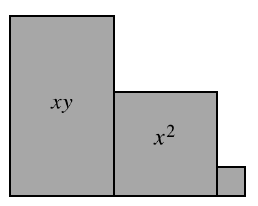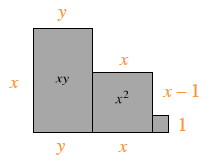### Home > CC3MN > Chapter 3 Unit 3 > Lesson CC3: 3.1.6 > Problem3-59

3-59.

Write an expression that represents the perimeter of the shape built with algebra tiles at right. Then find the perimeter if $x=3$ units and $y=7$ units. Homework Help ✎Find the length of each side, and then sum the lengths.

Once you find an expression for the perimeter, substitute $3$ for $x$ and $7$ for $y$.

$28$ units